## SOLVE your algebra problems step-by-step with MathPapa# MathPapa - Algebra Calculator

by MathPapa, Inc.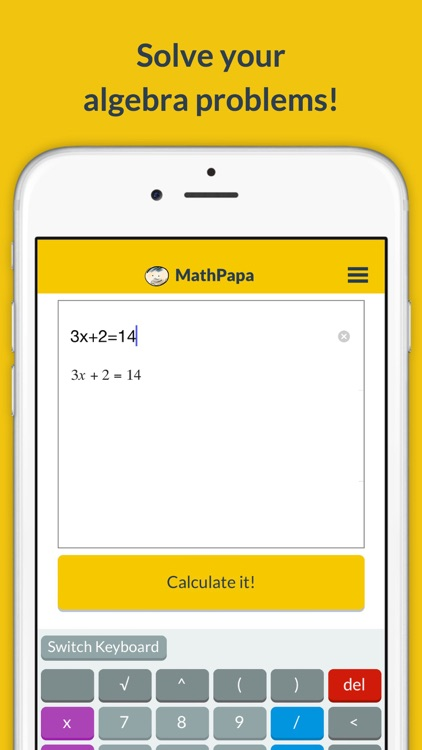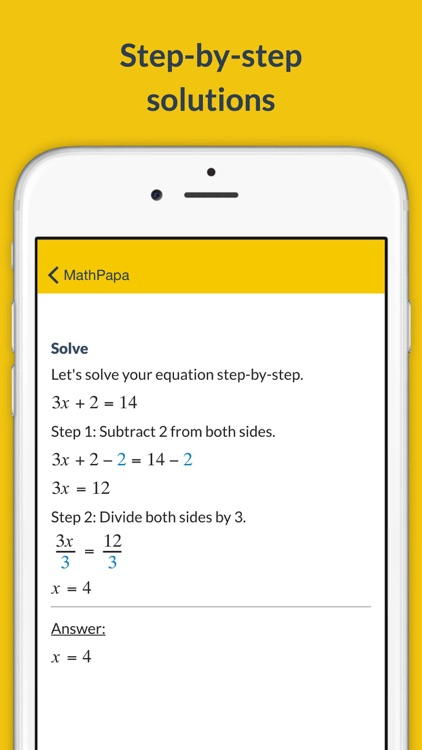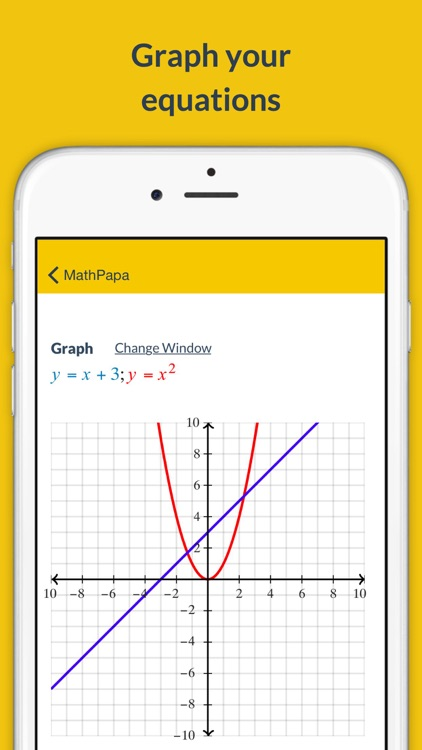SOLVE your algebra problems step-by-step with MathPapa!### App Details

Version
1.3.3
Rating
(7262)
Size
29Mb
Genre
Education Utilities
Last updated
November 6, 2018
Release date
August 31, 2015

• \$1.99

### App Screenshots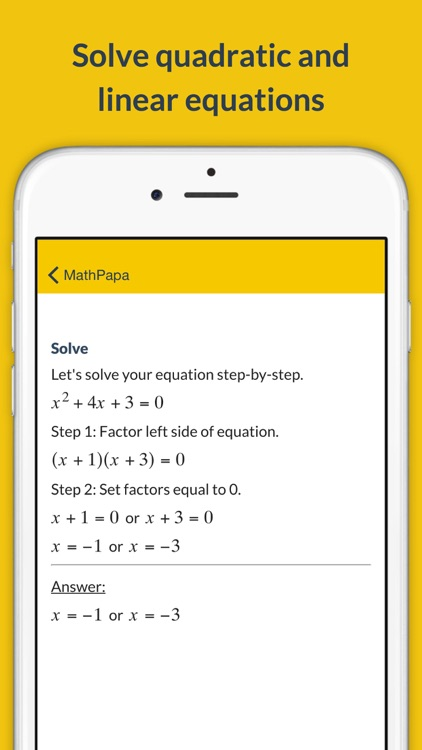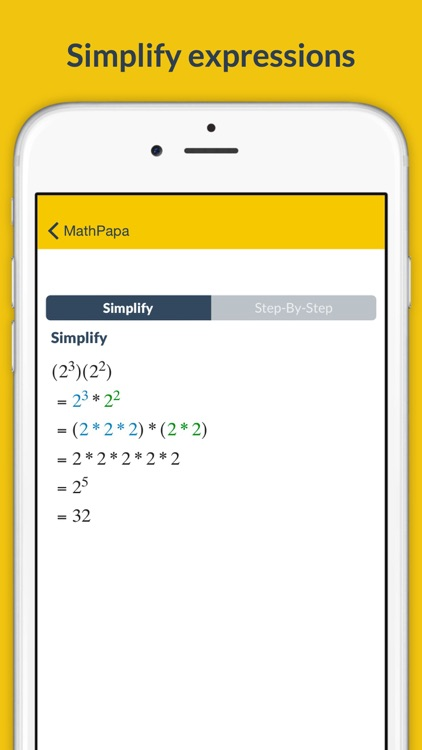### App Store Description

SOLVE your algebra problems step-by-step with MathPapa!

FEATURES:
• Solves linear equations and quadratic equations.
• Solves linear and quadratic inequalities.
• Graphs equations.
• Order of operations step-by-step.
• Evaluates expressions.
• Solves systems of two equations.

STEP-BY-STEP SOLUTIONS:

Get help on your algebra problems with the MathPapa Algebra Calculator!

HOW TO USE THE CALCULATOR:

Just type your problem into the text box.

For example, enter 3x+5=17 into the text box to get a step-by-step explanation of how to solve 3x+5=17.

MATH SYMBOLS:

Here are some symbols that the calculator understands:

- (Subtraction)
* (Multiplication)
/ (Division)
^ (Exponent: "raised to the power")
√ (Square Root)
|x| (Absolute Value of x)

For MathPapa Premium, there is a Monthly subscription for \$9.99.

This price is for U.S. customers. Pricing in other countries may vary.

Subscriptions will be charged via your iTunes account at the end of the free trial. Subscriptions will automatically renew unless canceled in Account Settings at least 24 hours before the end of the current period. Unused portion of Free Trial, if offered, is forfeited after purchase.